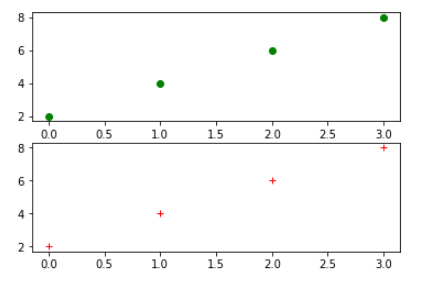# Draw subplots in matplotlib

python matplotlib 2 years, 1 month ago
import matplotlib.pyplot as plt plt.figure(1) plt.subplot(211) # the default plot properties applies plt.plot([2,4,6,8], 'go') plt.subplot(212) plt.plot([2,4,6,8], 'r+') plt.show()
414Instructions

You can see the output in the attached image.
If you want to play with plot then set specific properties in the plot function.
The basic plot is available here https://koderplace.com/code-samples/93/draw-a-basic-plot-using-matplotlib

### 0 Comments

Please Login to Comment Here